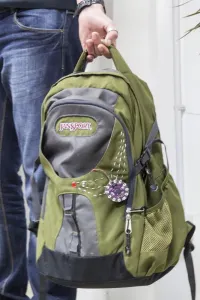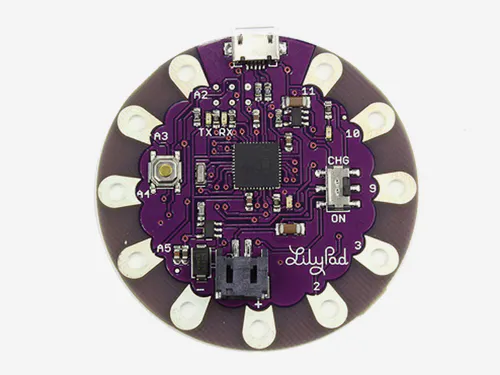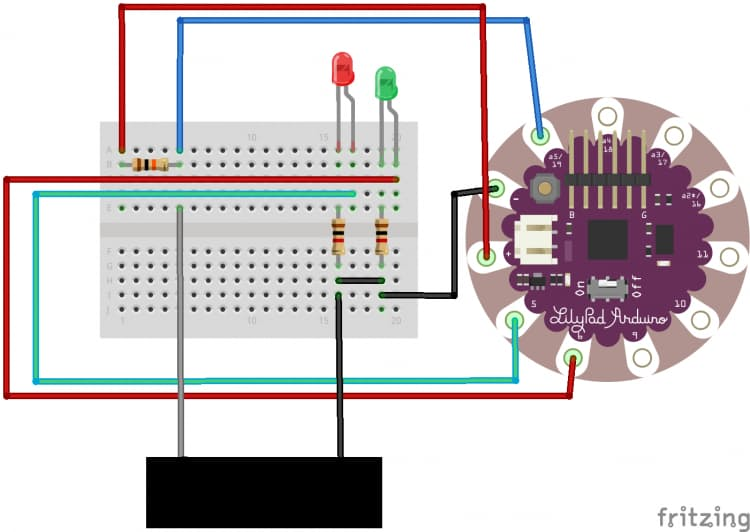Project tutorial# A Cool Scale © GPL3+

This tutorial aims to show how to make a cool scale for your bag using a lilypad and cool plug and wear stuff!

• 3,220 views
• 0 respects

## Components and supplies×1
 10 Red LED 5mm
×1
 10 Green LED 5mm
×1
 10 10k 1/4w resistor
×1
 10 1k 1/4w resistor
×1

### 1. Schematic

Build the simple circuits posted below! You can use standard AWG wire or the conductive cotton! As you prefer! The black box in the schematic represent the Textile Analog Pressure Sensor.

### 2. How it works

First of all you have to sew the Textile Analog Pressure Sensor on the handle of your bag (as shown below).

The Textile Analog Pressure Sensor is used in a voltage divider with a 10k resistor and the resulting voltage is read by the Arduino. The voltage divider is empirically calibrated to measure a weight range between 100g and 4kg.

### 3. Code

The working principle is very simple: based on an `analogRead` function two LEDs (Green and Red) are driven:

• the LEDs are both OFF with no load conditions;
• the Green LED lights up with a weight between 100g and 2kg;
• the LEDs are both ON with a weight between 2.1kg and 3kg;
• the LEDs are blinking over 3kg.
``````/*
Use your Arduino and your plug and wear accessories as a cool scale!
Working principle:
There are 2 LEDs (Green and Red):
- the LEDs are both OFF with no load conditions;
- the Green LED lights up with a weight between 100g and 2kg;
- the LEDs are both ON with a weight between 2.1kg and 3kg;
- the LEDs are blinking over 3kg
BOM:
Textile Analog Pressure Sensor
Conductive wire
1x 10k resistor
2x 1k resistor
1x Green LED
1x Red LED
Approximated reference table:
*/
const int no = 850;
const int ok = 530;
const int mmh = 410;
const int argh = 330;
const int sensor = A0;
const int greenLED = 5;
const int redLED = 6;
int analogVal;
void setup() {
// put your setup code here, to run once:
pinMode(sensor, INPUT);
pinMode(greenLED, OUTPUT); //green LED
pinMode(redLED, OUTPUT); //red LED
}
void loop() {
// put your main code here, to run repeatedly:
if (analogVal >= no) //no load
{
digitalWrite(greenLED, LOW);
digitalWrite(redLED, LOW);
}
if (analogVal >= ok && analogVal < no) //100g < weight < 2kg;
{
digitalWrite(greenLED, HIGH);
digitalWrite(redLED, LOW);
}
if (analogVal >= mmh && analogVal < ok) //2.1kg < weight < 3kg;
{
digitalWrite(greenLED, HIGH);
digitalWrite(redLED, HIGH);
}
if (analogVal <= argh) //weight > 3kg;
{
digitalWrite(greenLED, HIGH);
digitalWrite(redLED, HIGH);
delay(150);
digitalWrite(greenLED, LOW);
digitalWrite(redLED, LOW);
delay(150);
}
}
``````

## Code

##### Code snippet #1Arduino
```/*
Use your Arduino and your plug and wear accessories as a cool scale!
Working principle:
There are 2 LEDs (Green and Red):
- the LEDs are both OFF with no load conditions;
- the Green LED lights up with a weight between 100g and 2kg;
- the LEDs are both ON with a weight between 2.1kg and 3kg;
- the LEDs are blinking over 3kg

BOM:
Textile Analog Pressure Sensor
Conductive wire
1x 10k resistor
2x 1k resistor
1x Green LED
1x Red LED

Approximated reference table:

*/
const int no = 850;
const int ok = 530;
const int mmh = 410;
const int argh = 330;
const int sensor = A0;
const int greenLED = 5;
const int redLED = 6;
int analogVal;

void setup() {
// put your setup code here, to run once:
pinMode(sensor, INPUT);
pinMode(greenLED, OUTPUT); //green LED
pinMode(redLED, OUTPUT); //red LED
}

void loop() {
// put your main code here, to run repeatedly:

if (analogVal >= no) //no load
{
digitalWrite(greenLED, LOW);
digitalWrite(redLED, LOW);
}

if (analogVal >= ok && analogVal < no) //100g < weight < 2kg;
{
digitalWrite(greenLED, HIGH);
digitalWrite(redLED, LOW);
}

if (analogVal >= mmh && analogVal < ok) //2.1kg < weight < 3kg;
{
digitalWrite(greenLED, HIGH);
digitalWrite(redLED, HIGH);
}

if (analogVal <= argh) //weight > 3kg;
{
digitalWrite(greenLED, HIGH);
digitalWrite(redLED, HIGH);
delay(150);
digitalWrite(greenLED, LOW);
digitalWrite(redLED, LOW);
delay(150);
}

}
```

## SchematicsJuly 8, 2016

#### Members who respect this project

See similar projects
you might like

#### Weigh Objects with an Arduino Scale

Project showcase by Team Arduinotronics

• 80,578 views
• 43 respects

#### Arduino UNO High Precision Counting Scale

Project showcase by Fedeasche

• 18,931 views
• 47 respects

#### Digital Force Gauge & Weight Scale w/ Loadcell & Arduino

Project tutorial by ElectroPeak

• 34,741 views
• 9 respects

#### Analog Textile Press Button Tutorial

Project tutorial by Arduino_Scuola

• 2,658 views
• 8 respects

#### FizViz - Large Scale Physical Visualizations for Your Stats!

Project tutorial by Team IoT Design Shop

• 22,401 views